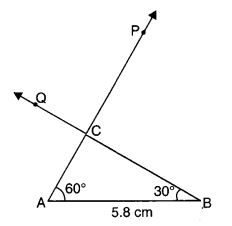NCERT Solutions for Class 7 Maths Chapter 10 Practical Geometry Ex 10.4 are part of NCERT Solutions for Class 7 Maths. Here we have given NCERT Solutions for Class 7 Maths Chapter 10 Practical Geometry Ex 10.4.

 Board CBSE Textbook NCERT Class Class 7 Subject Maths Chapter Chapter 10 Chapter Name Practical Geometry Exercise Ex 10.4 Number of Questions Solved 3 Category NCERT Solutions

## NCERT Solutions for Class 7 Maths Chapter 10 Practical Geometry Ex 10.4

Question 1.
Construct ∆ ABC, given m ∠A = 60°, m ∠B = 30° and AB = 5.8 cm.
Solution:
Steps of Construction1. Draw AB of length 5.8 cm.
2. At A, draw a ray AP making an angle of 60° with AB.
3. At B, draw a ray BQ making an angle of 30° with BA.
4. Mark the point of intersection of two rays as C.
5. ∆ ABC is now completed.

Question 2.
Construct ∆ PQR if PQ = 5 cm, m ∠PQR = 105° and m ∠QRP = 40°.
(Hint: Recall angle-sum property of a triangle).
Solution:
By angle-sum property of a triangle
m ∠RPQ + m ∠PQR + m ∠QRP = 180°
⇒ m ∠RPQ + 105° + 40° = 180°
⇒ m ∠RPQ + 145° = 180°
⇒ m ∠RPQ = 35°
Steps of Construction

1. Draw PQ of length 5 cm.
2. At Q, draw a ray QX making an angle of 105° with QP.3. At P draw a ray PY making an angle of 35° with PQ.
4. Mark the point of intersection of two rays as R.

∆ PQR is now completed.

Question 3.
Examine whether you can construct ∆DEF such that EF = 7.2 cm, m ∠E = 110° and m ∠F = 80°. Justify your answer.
Solution:
m ∠E + m ∠F = 110° + 80° = 190° > 180°
This is not possible since the sum of the measures of the three angles of a triangle is 180°. As such, the sum of two angles of a triangle cannot exceed 180°.
Hence, ∆ DEF cannot be constructed.

We hope the NCERT Solutions for Class 7 Maths Chapter 10 Practical Geometry Ex 10.4 helps you. If you have any query regarding NCERT Solutions for Class 7 Maths Chapter 10 Practical Geometry EX 10.4, drop a comment below and we will get back to you at the earliest.